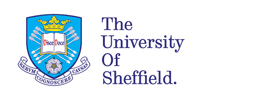Tony O'Hagan - Academic pages - Abstracts## Calculating Partial Expected Value of Perfect Information in Cost-Effectiveness Models

Alan Brennan, Samer Kharroubi, Anthony O’Hagan and Jim Chilcott

School of Health and Related Research and Department of Probability and Statistics, University of Sheffield, Sheffield, England

Publication details: Medical Decision Making 27, 448-470, 2007.

### Abstract

Partial EVPI calculations can quantify the value of learning about particular subsets of uncertain parameters in decision models. Published case studies have used different computational approaches. This paper aims to clarify computation of partial EVPI and encourage its use. Our mathematical description efining partial EVPI shows two nested expectations, which must be evaluated separately because of the need to compute a maximum between them. We set out a generalised Monte Carlo sampling algorithm using two nested simulation loops, firstly an outer loop to sample parameters of interest and only then an inner loop to sample the remaining uncertain parameters, given the sampled parameters of interest. Alternative computation methods and ‘shortcut’ algorithms are assessed and mathematical conditions for their use are considered. Maxima of Monte Carlo estimates of expectations are always biased upwards, and we demonstrate the effect of small samples on bias in computing partial EVPI. A series of case studies demonstrates the accuracy or otherwise of using ‘short-cut’ algorithm approximations in simple decision trees, models with increasingly correlated parameters, and many period Markov models with increasing non-linearity. The results show that even if relatively small correlation or non-linearity is present, then the ‘shortcut’ algorithm can be substantially inaccurate. The case studies also suggest that fewer samples on the outer level and larger numbers of samples on the inner level could be the most efficient approach to gaining accurate partial EVPI estimates. Remaining areas for methodological development are set out.